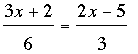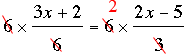SEARCH HOMEMath Central Quandaries & QueriesQuestion from Melissa, a student: I have a test tomorrow and I'm hoping you can help me before then. I can never seem to solve the "RESOLVE X" problems, or in french resous pour x/ They look like this. 3x+2\6=2x-5\3. I only understand NOTHING from that. Another equation is 2(x+1)=3(x+2). Please kindly help me. THANKS!Melissa,

For the second equation 2(x + 1) = 3(x + 2) the first step is to multiply through the parentheses.

2(x + 1) = 2x + 2 × 1 = 2x + 2 and
3(x + 2) = 3x + 3 × 2 = 3x + 6

Thus the equation becomes

2x + 2 = 3x + 6

You want to manipulate this equation, always maintaining the equality, and end with an equation of the form

x = some number.

Thus you want all the terms containing x on one side of the equation so add -3 to both sides. This maintains the equality and produces

2x + 2 - 3x = 3x + 6 - 3x or
2 - x = 6

You don't want the 2 on the left of the equality so add -2 to both sides

2 - x - 2 = 6 - 2 or
-x = 4

Finally multiply multiply both sides by -1 to get

(-1) × (-x) =(-1) × 4 or
x = -4

The first problem I assume isI would multiply both sides by 6 to obtainwhich becomes

3x + 2 = 2(2x - 5)

Now continue as in the second equation you sent.

Good luck on the test,
Penny

The English would be "Solve for X" or "Find X". (That may help make the goal clearer if English is your first language.)

Things you are allowed to do:

1. Multiply or divide both sides by the same thing

3(x+5) = 6(x-1) becomes x+5 = 2(x-1) (divide by 3)
(3x-1)/2 = 4 becomes 3x-1 = 8 (multiply by 2)

2. Add or subtract the same thing on both sides

x+3 = 2x -9 becomes 3 = x-9 (subtract x)
which becomes 12 = x (add 9)

3. Use the distributive law on one side or both

2(3x+5) = x becomes 6x + 15 = x (distributive law)
4x + 3x - 2 = 5 becomes 7x -2 = 5 (distributive law)

How you use these: first aim to get rid of parentheses and fractions with other operations "inside". Then try to get all terms with "x" onto one side and all terms without "x" onto the other. Thus:

(3x + 4)/2 = (7x-5)/3
(9x +12)/2 = 7x + 5 (multiply by 3)
9x + 12 = 14x + 10 (multiply by 2)
12 = 5x + 10 (subtract 9x)
2 = 5x (subtract 10)
2/5 = x (divide by 5)

-RDMath Central is supported by the University of Regina and The Pacific Institute for the Mathematical Sciences.